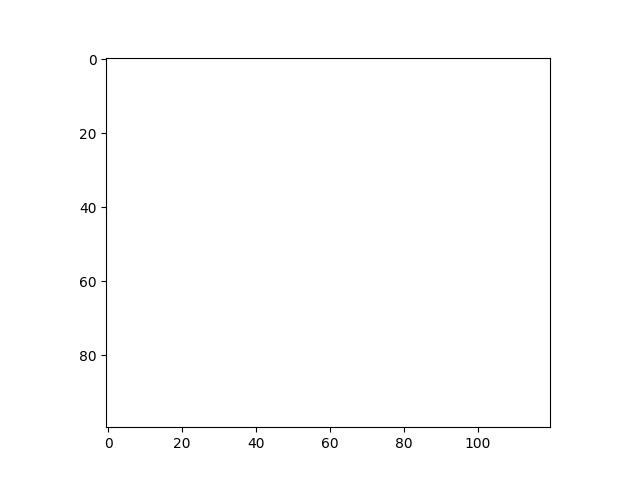# Animated image using a precomputed list of images¶```import numpy as np
import matplotlib.pyplot as plt
import matplotlib.animation as animation

fig = plt.figure()

def f(x, y):
return np.sin(x) + np.cos(y)

x = np.linspace(0, 2 * np.pi, 120)
y = np.linspace(0, 2 * np.pi, 100).reshape(-1, 1)
# ims is a list of lists, each row is a list of artists to draw in the
# current frame; here we are just animating one artist, the image, in
# each frame
ims = []
for i in range(60):
x += np.pi / 15.
y += np.pi / 20.
im = plt.imshow(f(x, y), animated=True)
ims.append([im])

ani = animation.ArtistAnimation(fig, ims, interval=50, blit=True,
repeat_delay=1000)

# To save the animation, use e.g.
#
# ani.save("movie.mp4")
#
# or
#
# from matplotlib.animation import FFMpegWriter
# writer = FFMpegWriter(fps=15, metadata=dict(artist='Me'), bitrate=1800)
# ani.save("movie.mp4", writer=writer)

plt.show()
```

Gallery generated by Sphinx-Gallery